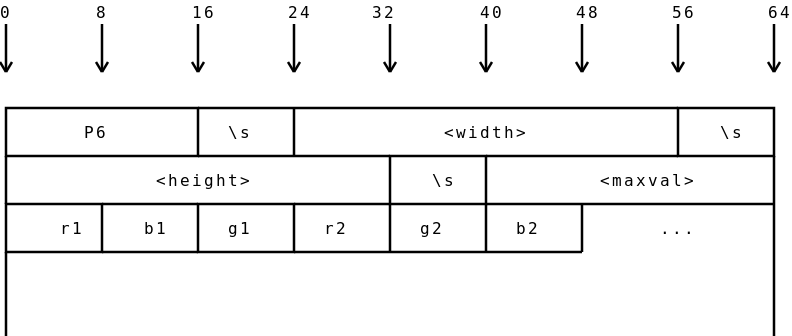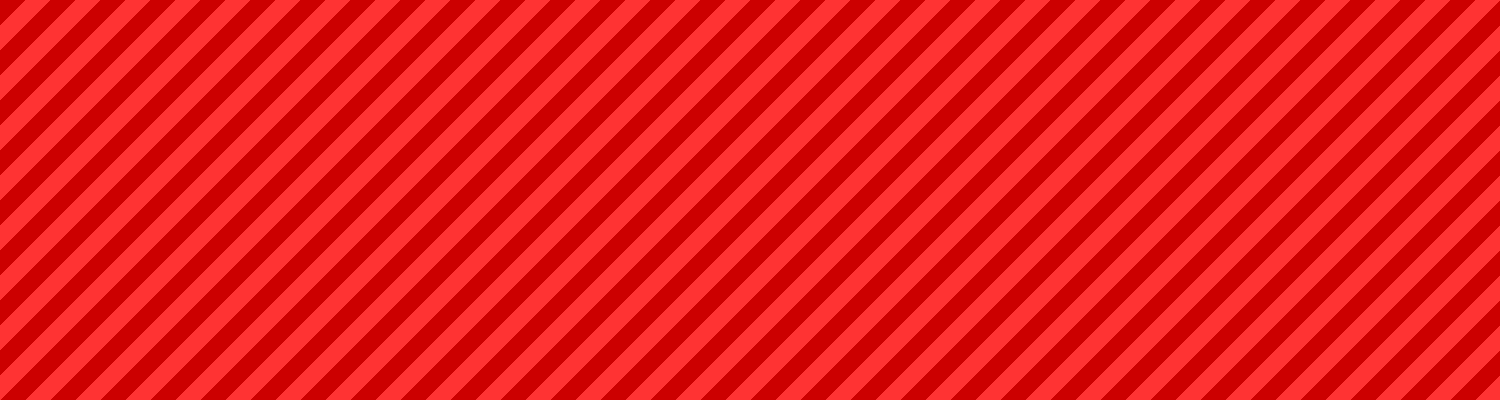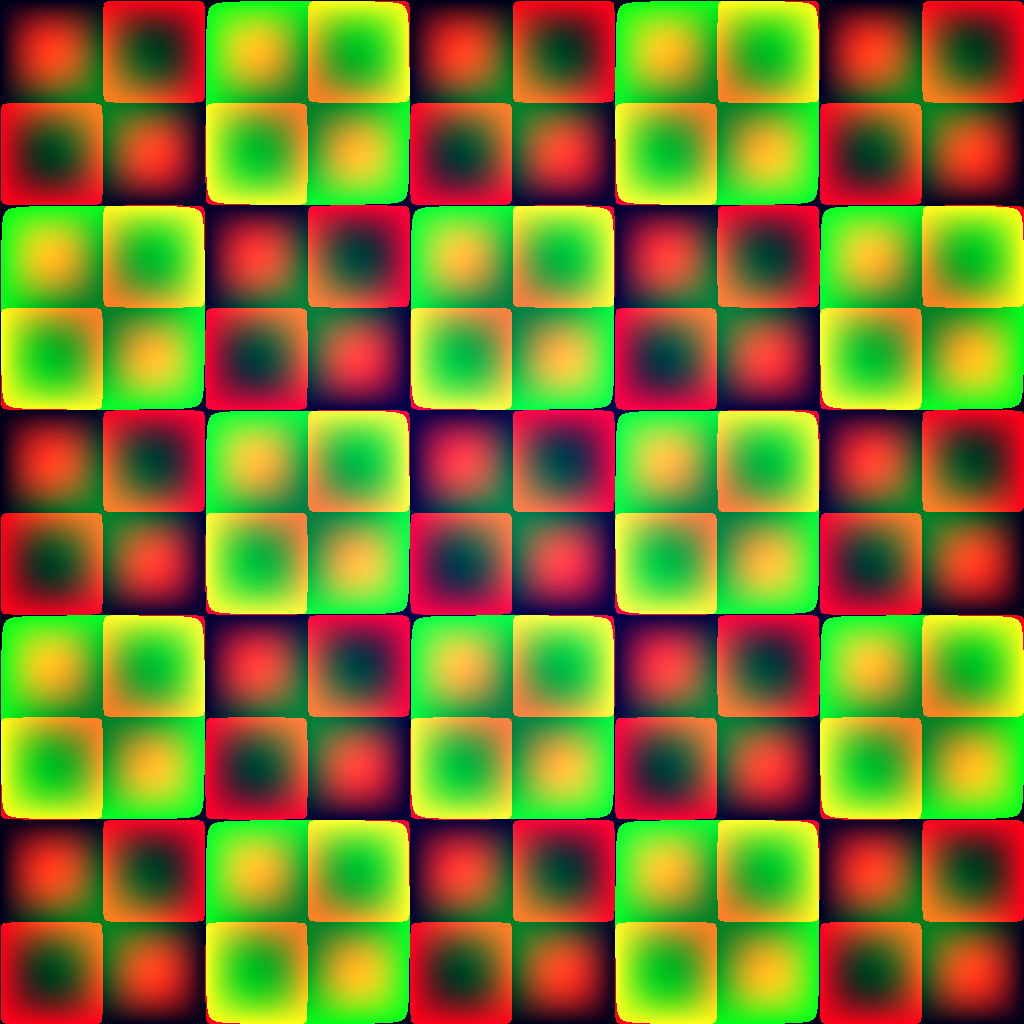Making Mathematical Art with C Sharp and PPM

The other day I wanted to (for some random reason) create some stripes. Having worked out a simple algorithm that would produce some rather nice stripes given an (x, y) pixel coordinate, I set out to write a small script that would generate some stripes for me.

I discovered that it wasn't as easy as I'd thought. Lockbits confused me, and I couldn't find a good enough example to learn from. Thankfully I caught wind of a ridiculously simple image format called PPM (Portable Pixel Map) that I could use to output a byte[] array of pixel data as a valid image.

Here's a diagram I made to illustrate the format:The format basically consists of a ascii header, followed by a raw dump of a byte[] full of pixel data. The header contains several parts:

1. The characters P6 (This is called the 'magic byte', and can be used to identify the type of content that a file contains)
2. A single whitespace (I used \s in the diagram because that is the escape code for whitespace in a javascript regular expression)
3. The width of the image, in ascii
4. Another single whitespace
5. The height of the image, in ascii
6. Another single whitespace
7. The maximum value that the red / green / blue pixels will go up to. This value will be considered 100% saturated. Normally, you'd want this to be 255.
8. Another single whitespace - not shown on the diagram (oops); usually a new line (\n).
9. The raw byte[] array of pixel data.

Once you've your pixel data as a PPM, you can then use something like imagemagick to convert it to a png with a command like mogrify -format png image.ppm or convert image.ppm image.png.Using this method, you can generate almost anything using pure C#. Here's the code I used to generate the above stripes:

using System;
using System.IO;

public class EmptyClass
{
#region Settings

static string filename = "image.ppm";

static int width = 1500;
static int height = 400;

static int stripeWidth = width / 30;
static rgb stripeLowCol = new rgb(204, 0, 0);
static rgb stripeHighCol = new rgb(255, 51, 51);

static float multiplier = 1f;

#endregion

#region Image Generator

public static void Main()
{
byte[] pixelData = new byte[width * height * 3];
for(int x = 0; x < width; ++x)
{
for(int y = 0; y < height; ++y)
{
int currentPixel = ((y * width) + x) * 3;
pixelData[currentPixel] = redPixel(x, y);
pixelData[currentPixel + 1] = greenPixel(x, y);
pixelData[currentPixel + 2] = bluePixel(x, y);
}
}

StreamWriter destination = new StreamWriter(filename);
destination.Write("P6\n{0} {1}\n{2}\n", width, height, 255);
destination.Flush();
destination.BaseStream.Write(pixelData, 0, pixelData.Length);
destination.Close();
}

#endregion

#region Pixel value functions - edit these

public static byte redPixel(int x, int y)
{
return (byte)(((x + y) % stripeWidth < stripeWidth / 2 ? stripeLowCol.r : stripeHighCol.r) * multiplier);
}
public static byte greenPixel(int x, int y)
{
return (byte)(((x + y) % stripeWidth < stripeWidth / 2 ? stripeLowCol.g : stripeHighCol.g) * multiplier);
}
public static byte bluePixel(int x, int y)
{
return (byte)(((x + y) % stripeWidth < stripeWidth / 2 ? stripeLowCol.b : stripeHighCol.b) * multiplier);
}

#endregion
}

#region Utility Classes
class rgb
{
public byte r, g, b;
public rgb(byte inCol)
{
r = g = b = inCol;
}
public rgb(byte inR, byte inG, byte inB)
{
r = inR;
g = inG;
b = inB;
}
}
#endregion

The settings at the top control the appearance of the output. filename is the filename to write the image to, width and height set the dimensions of the image, stripeWidth sets the width in pixels of each stripe, and stripeLowCol and stripeHighCol set the colour of the different stripes. The multiplier at the end isn't actually needed, but you can use it to brighten or dim the resulting image if you want.

Not content with stripes, I played around for a bit longer and came up with this:Above: My second attempt at mathematical art. It looks better in my native image previewer...

The above actually consists of a 3 different functions - one for each channel. Here they are:

public static byte redPixel(int x, int y)
{
return (byte)(Math.Sin(x / (width / (Math.PI * 10))) * 255 * Math.Sin(y / (height / (Math.PI*10))));
}
public static byte greenPixel(int x, int y)
{
return (byte)(Math.Sin(x / (width / (Math.PI * 5))) * 128 * Math.Sin(y / (height / (Math.PI * 5))));
}
public static byte bluePixel(int x, int y)
{
return (byte)((Math.Sin(x / (width / Math.PI)) * 52 * Math.Sin(y / (height / Math.PI))) + 25);
}

I don't actually know how it works (even though I wrote it strangely enough), but if you do know, please leave a comment down below!

Since it might be a bit difficult to see, here's an animated gif that shows each of the colour channels broken down:Lastly, I have rendered a larger copy of the above. You can view it here (Size: 4.8MB).

Have you made some interesting mathematical art? Post it in the comments below!

Tag Cloud

3d 3d printing account algorithms android announcement architecture archives arduino artificial intelligence artix assembly async audio automation backups bash batch blog bookmarklet booting bug hunting c sharp c++ challenge chrome os code codepen coding conundrums coding conundrums evolved command line compilers compiling compression css dailyprogrammer data analysis debugging demystification distributed computing documentation downtime electronics email embedded systems encryption es6 features ethics event experiment external first impressions future game github github gist gitlab graphics hardware hardware meetup holiday holidays html html5 html5 canvas infrastructure interfaces internet interoperability io.js jabber jam javascript js bin labs learning library linux lora low level lua maintenance manjaro network networking nibriboard node.js operating systems own your code performance phd photos php pixelbot portable privacy problem solving programming problems project projects prolog protocol protocols pseudo 3d python reddit redis reference releases resource review rust searching secrets security series list server software sorting source code control statistics storage svg talks technical terminal textures thoughts three thing game three.js tool tutorial twitter ubuntu university update updates upgrade version control virtual reality virtualisation visual web website windows windows 10 xmpp xslt

Art by Mythdael